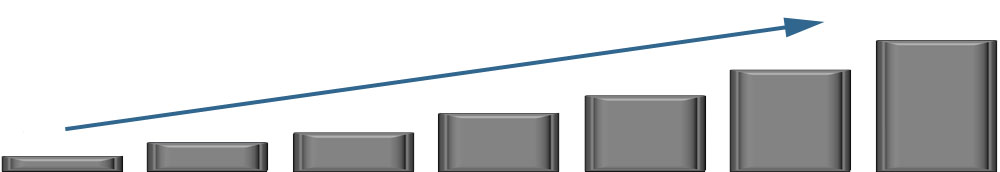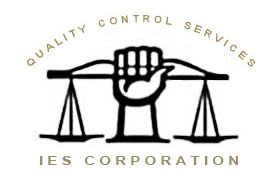﻿ IES Corporation ( IES/QCS ) - Linearity Test

# QCS / IES Corporation

2324 SE 11th Ave Portland, OR 97214
800.541.0852Linearity:

## Linearity Test:

Perhaps the most obvious test of a high precision scale would be to place a weight of accurately known value on the weighing pan, and observe the numerical result. But there is a better test, nearly as simple, that better reflects the measurement accuracy. This is called the linearity test.

The linearity test measures the ability of an instrument to have consistent sensitivity throughout the weighing range. The test requires several nominally equal weights, each a fraction of the weighing capacity. The group together should approximate the weighing range of the instrument. For example, a 160 gram capacity analytical balance might be tested with three 50 gram weights.

The test weights must be distinguishable from each other, for example labeled A, B, and C. Here is the procedure:

1) Tare the unit to read all zeroes.

2) Weigh test weight A, record the result, then remove A

3) Place weight B on the pan , then tare to zeroes.

4) Place weight A on the pan, record the result, then remove A.

5) Place weight C on the pan with B, then tare to zeroes.

6) Place weight A on the pan, and record the result.

7) Compare the readings recorded at steps 2, 4, and 6. These should be consistent within the specifications of the instrument. Since the same item is being weighed each time, the readings should be the same, within the accuracy specifications of the instrument. The group of weights as a whole can be used for full-scale calibration.### Shop Tip:

Substitution Linearity:

Linearity testing is one of the top question we receive. The point of subsitution linearity is to remove any errors in weights, so can be done no matter the class of weight being used or the resolution of the unit.

Simply weigh a single object, with varying amounts of preload.

RMA - Repair Initiation
office@iescorp.com